# Nuclear Physics MSQ

## 10 Questions MCQ Test Modern Physics for IIT JAM | Nuclear Physics MSQ

Description
This mock test of Nuclear Physics MSQ for Physics helps you for every Physics entrance exam. This contains 10 Multiple Choice Questions for Physics Nuclear Physics MSQ (mcq) to study with solutions a complete question bank. The solved questions answers in this Nuclear Physics MSQ quiz give you a good mix of easy questions and tough questions. Physics students definitely take this Nuclear Physics MSQ exercise for a better result in the exam. You can find other Nuclear Physics MSQ extra questions, long questions & short questions for Physics on EduRev as well by searching above.
*Multiple options can be correct
QUESTION: 1

### For a radioactive substance with half life t1/2 and initial number of nuclei N0. The number the nuclei decayed in 3 half lives is :

Solution:

Number of nuclei remaining after
n half lives is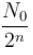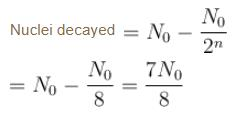The correct answer is: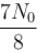*Multiple options can be correct
QUESTION: 2

### A singly charged positive ion is accelerated through a potential difference of 1000V in a mass spectrograph. It then passes through a uniform magnetic field B = 1500 Gauss and then deflected into a circular path of radius 0.122 m.  Select the correct statements

Solution: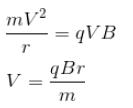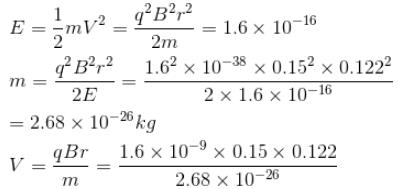= 1.09 × 105 m/s

The correct answers are: The speed of the ion is 1.09 × 105 m/s, The mass of the ion is 2.68 × 10–26 kg

*Multiple options can be correct
QUESTION: 3

### A singly charged positive ion is accelerated through a potential difference of 1000V in a mass spectrograph. It then passes through a uniform magnetic field B = 1500 Gauss and then deflected into a circular path of radius 0.122 m. The mass number of the ion is :

Solution:

Mass No. = No. of p + No. of neutron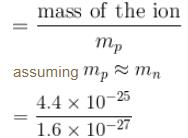= 2.75 × 102
A = 275

*Multiple options can be correct
QUESTION: 4

Choose the correct option for mirror nuclei

Solution:

Mirror nuclei have same A but the number of neutrons & protons are reversed.
There is a slight difference in the masses of a proton & neutron. This apart from the different coulomb force in mirror nuclei result in the difference in their masses.

The correct answers are: Nuclei with same mass number but the number of neutrons and protons reversed are called mirror nuclei, The mirror nuclei have different masses, The difference in masses is due to the difference in the proton & neutron masses & difference in the coulomb energy.

*Multiple options can be correct
QUESTION: 5

1 mg of a radioactive material with half life 1600 years is kept for 2000 years. Choose the correct option.

Solution: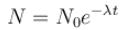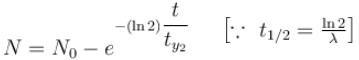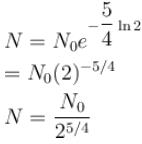mass remaining after 2000 years is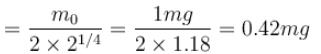mass decayed = 0.58 mg

The correct answers are: 0.42 mg  material was left after 2000 years, 0.58 mg  material decayed in 2000 years

*Multiple options can be correct
QUESTION: 6

What are the reasons leading to the discovery of a neutrino in the case of a Beta decay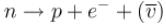Solution:

The correct answers are: Continuous βray spectrum instead of a discrete spectrum as would have been the case had there only been an electron emitted, Most electrons were emitted with energy much less than maximum kinetic energy., Non conservation of angular momentum, Non conservation of linear momentum

*Multiple options can be correct
QUESTION: 7

Nuclear force is

Solution:

The correct answers are: spin dependent, charge independent, has repulsive core, same between two proton and two neutron

*Multiple options can be correct
QUESTION: 8

From the Binding energy per nucleon curve, one can deduce that

Solution:

B.E./Neutron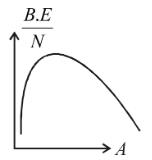Nuclei with smaller A fuse to form a nucleus of higher A which has more B.E./nucleon compared to the reactants. Nuclei with higher A(~200) fission into nuclei of smaller A which have higher B.E./nuclei  (thus they are more stable).

The correct answers are: Nuclei with lower mass number tend to undergo fusion, Nuclei with larger mass number tend to undergo nuclear fission

*Multiple options can be correct
QUESTION: 9

Which of the following is true about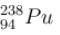Solution:

Deuteron emission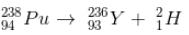α particle emission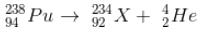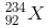has a lower mass number & from the B.E/nucleon v/s A plot, it can be concluded that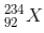will have a greater B.E./nucleon as compared to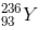∴  it would be more stable
∴  α decay is preferred

The correct answers are: 238Pu prefers to decay by emitting an α particle., Emitting an α particle results in a nucleus having greater binding energy per nucleon.

*Multiple options can be correct
QUESTION: 10

Which of the following is true regarding the beam energies required to probe the inside of nucleus

Solution:

For an electron beam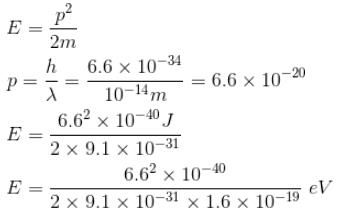~0.22 × 1010
= 2.2 × 109 eV
= 2.2 GeV
For proton beams,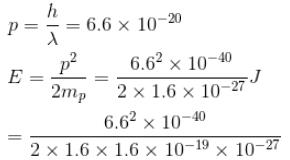= 8.5 × 106 eV

= 8.5 MeV

The correct answers are: The energy of a proton beam to probe the inside of a nucleus is of order MeV., The energy of an electron beam to probe the inside of a nucleus is of the order of GeV.